Dr. S. R. Lasker Library Online Catalogue

Normal view

# Statistical modelling in R / Murray Aitkin ... [et al.].

Material type:TextLanguage: English Series: Oxford statistical science series ; 35Publication details: 2009. Description: xii, 576 p. : ill. ; 24 cmISBN: 9780199219131 (pbk); 0199219133 (pbk); 9780199219148 (hbk); 0199219141 (hbk)DDC classification: 519.502855133 LOC classification: QA276.45.R3 | S73 2009Online resources: Table of contents only | WorldCat details
Contents:
TOC INTRODUCING R: Statistical packages and statistical modelling -- Getting started in R -- Reading data into R -- Assignment and data generation -- Displaying data -- Data structures and the workspace -- Transformations and data modification -- Functions and suffixing -- Structure functions -- Mathematical functions -- Logical operators -- Control functions -- Statistical functions -- Random numbers -- Suffixes in expressions -- Extracting subsets of data -- Recoding variates and factors into new factors -- Graphical facilities -- Text functions -- Writing your own functions -- Sorting and tabulation -- Editing R code -- Installing and using packages -- STATISTICAL MODELLING AND INFERERENCE: Statistical models -- Types of variables -- Population models -- Random sampling -- The likelihood function -- Inference for single parameter models -- Comparing two simple hypotheses -- Information about a single parameter -- Comparing a simple null hypothesis and a composite alternative -- Inference with nuisance parameters -- Profile likelihoods -- Marginal likelihood for the variance -- Likelihood normalizing transformations -- Alternative test procedures -- Bayes inference -- Binomial model -- Hypergeometric sampling from finite populations -- The effect of the sample design on inference -- The exponential family -- Mean and variance -- Generalized linear models -- Maximum likelihood fitting of the GLM -- Model comparisons through maximized likelihoods -- Likelihood inference without models -- Likelihoods for percentiles -- Empirical likelihood. REGRESSION AND ANALYSIS OF VARIANCE: An example -- Strategies for model simplification -- Stratified, weighted and clustered samples -- Model criticism -- Mis-specification of the probability distribution -- Mis-specification of the link function -- The occurrence of aberrant and influential observations -- Mis-specification of the systematic part of the model -- The Box-Cox transformation family -- Modelling and background information -- Link functions and transformations -- Regression models for prediction -- Model choice and mean square prediction error -- Model selection through cross-validation -- Reduction of complex regression models -- Sensitivity of the Box-Cox transformation -- The use of regression models for calibration -- Measurement error in the explanatory variables -- Factorial designs -- Unbalanced cross-classifications -- The Bennett hostility data -- ANOVA of the cross-classification -- Regression analysis of the cross-classification -- Statistical package treatments of cross-classifications -- Missing data -- Approximate methods for missing data -- Modelling of variance heterogeneity -- Poison example -- Tree example. BINARY RESPONSE DATA: Binary responses -- Transformations and link functions -- Profile likelihoods for functions of parameters -- Model criticism -- Mis-specification of the probability distribution -- Mis-specification of the link function -- The occurrence of aberrant and influential observations -- Binary data with continuous covariates -- Contingency table construction from binary data -- The prediction of binary outcomes -- Profile and conditional likelihoods in 2 x 2 tables -- Three-dimensional contingency tables with a binary response -- Prenatal care and infant mortality -- Coronary heart disease -- Multidimensional contingency tables with a binary response -- MULTINOMIAL AND POISSON RESPONSE DATA: The Poisson distribution -- Cross-classified counts -- Multicategory responses -- Multinomial logit model -- The Poisson-multinomial relation -- Fitting the multinomial logit model -- Ordered response categories -- Common slopes for the regressions -- Linear trend over response categories -- Proportional slopes -- The continuation ratio model -- Other models -- An Example -- Multinomial logit model -- Continuation ratio model -- Structured multinomial responses -- Independent outcomes -- Correlated outcomes. SURVIVAL DATA: Introduction -- The exponential distribution -- Fitting the exponential distribution -- Model criticism -- Comparison with the normal family -- Censoring -- Likelihood function for censored observations -- Probability plotting with censored data: the Kaplan-Meier estimator -- The gamma distribution -- Maximum likelihood with uncensored data -- Maximum likelihood with censored data -- Double modelling -- The Weibull distribution -- Maximum likelihood fitting of the Weibull distribution -- The extreme value distribution -- The reversed extreme value distribution -- Survivor function plotting for the Weibull and extreme value distributions -- The Cox proportional hazards model and the piecewise exponential distribution -- Maximum likelihood fitting of the piecewise exponential distribution -- Examples -- The logistic and log-logistic distributions -- The normal and lognormal distributions -- Evaluating the proportional hazard assumption -- Competing risks -- Time-dependent explanatory variables -- Discrete time models -- FINITE MIXTURE MODELS: Introduction -- Example -- girl birthweights -- Finite mixtures of distributions -- Maximum likelihood in finite mixtures -- Standard errors -- Testing for the number of components -- Example -- Likelihood 'spikes' -- Galaxy data -- Kernel density estimates. RANDOM EFFECT MODELS : Overdispersion -- Testing for overdispersion -- Conjugate random effects -- Normal kernel: the t-distribution -- Poisson kernel: the negative binomial distribution -- Binomial kernel: beta-binomial distribution -- Gamma kernel -- Difficulties with the conjugate approach -- Normal random effects -- Predicting from the normal random effect model -- Gaussian quadrature examples -- Overdispersion model fitting -- Poisson -- the fabric fault data -- Binomial -- the toxoplasmosis data -- Other specified random effect distributions -- Arbitrary random effects -- Examples -- The fabric fault data -- The toxoplasmosis data -- Leukaemia remission data -- The Brownlee stack-loss data -- Random coefficient regression models -- Example -- the fabric fault data -- Algorithms for mixture fitting -- The trypanosome data -- Modelling the mixing probabilities -- Mixtures of mixtures -- VARIANCE COMPONENT MODELS: Models with shared random effects -- The normal/normal model -- Exponential family two-level models -- Other approaches -- NPML estimation of the masses and mass-points -- Random coefficient models -- Variance component model fitting -- Children's height development -- Multi-centre trial of beta-blockers -- Longitudinal study of obesity -- Autoregressive random effect models -- Latent variable models -- The normal factor model -- IRT models -- The Rasch model -- The two-parameter model -- The three-parameter logit (3PL) model -- Example -- The Law School Aptitude Test (LSAT) -- Spatial dependence -- Multivariate correlated responses -- Discreteness of the NPML estimate.
Summary: "R is now the most widely used statistical package/language in university statistics departments and many research organisations. Its great advantages are that it is now the leading-edge statistical package/language and that it can be freely downloaded from the R website. Its cooperative development and open code also attract many contributors which means that the modelling and data analysis possibilities in R are
Tags from this library: No tags from this library for this title.
Star ratingsAverage rating: 0.0 (0 votes)
Holdings
Item type Current library Collection Call number Copy number Status Date due Barcode Item holdsText
Reserve Section
Non-fiction 519.502855133 STA 2009 (Browse shelf(Opens below)) C-1 Not For Loan 27306Text
Circulation Section
Non-fiction 519.502855133 STA 2009 (Browse shelf(Opens below)) C-2 Available 27307
Total holds: 0
##### Browsing EWU Library shelves, Shelving location: Reserve Section Close shelf browser (Hides shelf browser)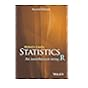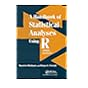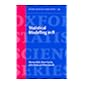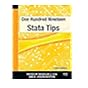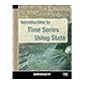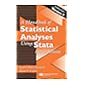519.502855133 CRS 2015 Statistics : 519.502855133 HOH 2014 A handbook of statistical analyses using R / 519.502855133 HOH 2014 A handbook of statistical analyses using R / 519.502855133 STA 2009 Statistical modelling in R / 519.5028553 ONE 2014 One hundred nineteen stata tips / 519.50285536 BEI 2013 Introduction to time series using Stata / 519.50285536 HEH 2007 A handbook of statistical analyses using Stata /

Includes bibliographical references (p. -565) and indexes.

TOC INTRODUCING R: Statistical packages and statistical modelling --
Getting started in R --
Assignment and data generation --
Displaying data --
Data structures and the workspace --
Transformations and data modification --
Functions and suffixing --
Structure functions --
Mathematical functions --
Logical operators --
Control functions --
Statistical functions --
Random numbers --
Suffixes in expressions --
Extracting subsets of data --
Recoding variates and factors into new factors --
Graphical facilities --
Text functions --
Sorting and tabulation --
Editing R code --
Installing and using packages --
STATISTICAL MODELLING AND INFERERENCE: Statistical models --
Types of variables --
Population models --
Random sampling --
The likelihood function --
Inference for single parameter models --
Comparing two simple hypotheses --
Information about a single parameter --
Comparing a simple null hypothesis and a composite alternative --
Inference with nuisance parameters --
Profile likelihoods --
Marginal likelihood for the variance --
Likelihood normalizing transformations --
Alternative test procedures --
Bayes inference --
Binomial model --
Hypergeometric sampling from finite populations --
The effect of the sample design on inference --
The exponential family --
Mean and variance --
Generalized linear models --
Maximum likelihood fitting of the GLM --
Model comparisons through maximized likelihoods --
Likelihood inference without models --
Likelihoods for percentiles --
Empirical likelihood. REGRESSION AND ANALYSIS OF VARIANCE: An example --
Strategies for model simplification --
Stratified, weighted and clustered samples --
Model criticism --
Mis-specification of the probability distribution --
Mis-specification of the link function --
The occurrence of aberrant and influential observations --
Mis-specification of the systematic part of the model --
The Box-Cox transformation family --
Modelling and background information --
Regression models for prediction --
Model choice and mean square prediction error --
Model selection through cross-validation --
Reduction of complex regression models --
Sensitivity of the Box-Cox transformation --
The use of regression models for calibration --
Measurement error in the explanatory variables --
Factorial designs --
Unbalanced cross-classifications --
The Bennett hostility data --
ANOVA of the cross-classification --
Regression analysis of the cross-classification --
Statistical package treatments of cross-classifications --
Missing data --
Approximate methods for missing data --
Modelling of variance heterogeneity --
Poison example --
Tree example. BINARY RESPONSE DATA: Binary responses --
Profile likelihoods for functions of parameters --
Model criticism --
Mis-specification of the probability distribution --
Mis-specification of the link function --
The occurrence of aberrant and influential observations --
Binary data with continuous covariates --
Contingency table construction from binary data --
The prediction of binary outcomes --
Profile and conditional likelihoods in 2 x 2 tables --
Three-dimensional contingency tables with a binary response --
Prenatal care and infant mortality --
Coronary heart disease --
Multidimensional contingency tables with a binary response --
MULTINOMIAL AND POISSON RESPONSE DATA: The Poisson distribution --
Cross-classified counts --
Multicategory responses --
Multinomial logit model --
The Poisson-multinomial relation --
Fitting the multinomial logit model --
Ordered response categories --
Common slopes for the regressions --
Linear trend over response categories --
Proportional slopes --
The continuation ratio model --
Other models --
An Example --
Multinomial logit model --
Continuation ratio model --
Structured multinomial responses --
Independent outcomes --
Correlated outcomes. SURVIVAL DATA: Introduction --
The exponential distribution --
Fitting the exponential distribution --
Model criticism --
Comparison with the normal family --
Censoring --
Likelihood function for censored observations --
Probability plotting with censored data: the Kaplan-Meier estimator --
The gamma distribution --
Maximum likelihood with uncensored data --
Maximum likelihood with censored data --
Double modelling --
The Weibull distribution --
Maximum likelihood fitting of the Weibull distribution --
The extreme value distribution --
The reversed extreme value distribution --
Survivor function plotting for the Weibull and extreme value distributions --
The Cox proportional hazards model and the piecewise exponential distribution --
Maximum likelihood fitting of the piecewise exponential distribution --
Examples --
The logistic and log-logistic distributions --
The normal and lognormal distributions --
Evaluating the proportional hazard assumption --
Competing risks --
Time-dependent explanatory variables --
Discrete time models --
FINITE MIXTURE MODELS: Introduction --
Example --
girl birthweights --
Finite mixtures of distributions --
Maximum likelihood in finite mixtures --
Standard errors --
Testing for the number of components --
Example --
Likelihood 'spikes' --
Galaxy data --
Kernel density estimates. RANDOM EFFECT MODELS : Overdispersion --
Testing for overdispersion --
Conjugate random effects --
Normal kernel: the t-distribution --
Poisson kernel: the negative binomial distribution --
Binomial kernel: beta-binomial distribution --
Gamma kernel --
Difficulties with the conjugate approach --
Normal random effects --
Predicting from the normal random effect model --
Overdispersion model fitting --
Poisson --
the fabric fault data --
Binomial --
the toxoplasmosis data --
Other specified random effect distributions --
Arbitrary random effects --
Examples --
The fabric fault data --
The toxoplasmosis data --
Leukaemia remission data --
The Brownlee stack-loss data --
Random coefficient regression models --
Example --
the fabric fault data --
Algorithms for mixture fitting --
The trypanosome data --
Modelling the mixing probabilities --
Mixtures of mixtures --
VARIANCE COMPONENT MODELS: Models with shared random effects --
The normal/normal model --
Exponential family two-level models --
Other approaches --
NPML estimation of the masses and mass-points --
Random coefficient models --
Variance component model fitting --
Children's height development --
Multi-centre trial of beta-blockers --
Longitudinal study of obesity --
Autoregressive random effect models --
Latent variable models --
The normal factor model --
IRT models --
The Rasch model --
The two-parameter model --
The three-parameter logit (3PL) model --
Example --
The Law School Aptitude Test (LSAT) --
Spatial dependence --
Multivariate correlated responses --
Discreteness of the NPML estimate.

"R is now the most widely used statistical package/language in university statistics departments and many research organisations. Its great advantages are that it is now the leading-edge statistical package/language and that it can be freely downloaded from the R website. Its cooperative development and open code also attract many contributors which means that the modelling and data analysis possibilities in R are

AS

Sagar Shahanawaz

There are no comments on this title.